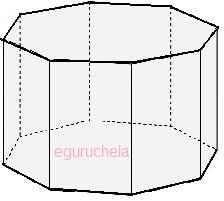# Octogonal prism Area, Volume and Surface Area calculator

Calculates the octagonal-prism area, octagonal-prism volume and octagonal-prism surface area for given side length(a), height(l) and distance(d).

 Area (A) Volume (V) Surface Area (SA)

### formulas:

 Area (A) 2 X side-length(a) X distance(d) Volume (V) Area(A) X height(l) Surface Area (SA) (2 X A) + (8 X a X l)The octagonal prism is solid (3-dimensional) object having

total 10 faces, 24 edges and 16 vertices.

The octagonal prism has rectangle side shape and octagonal shape for top & base.